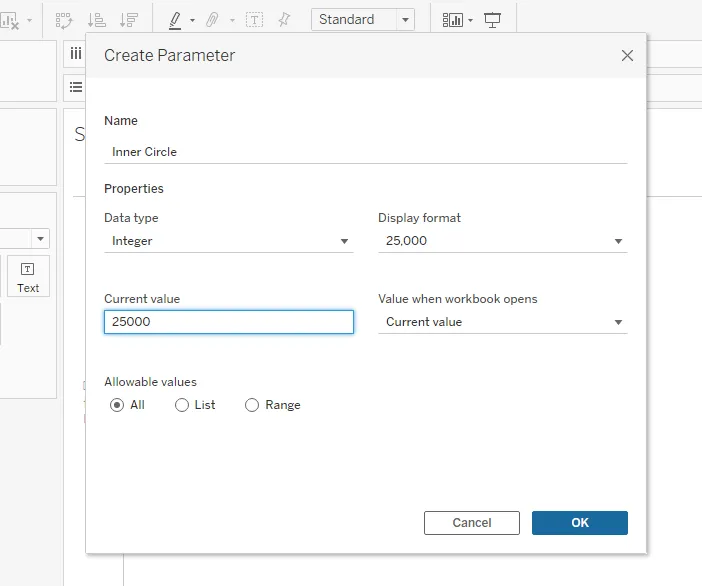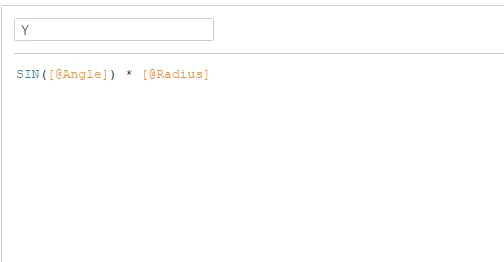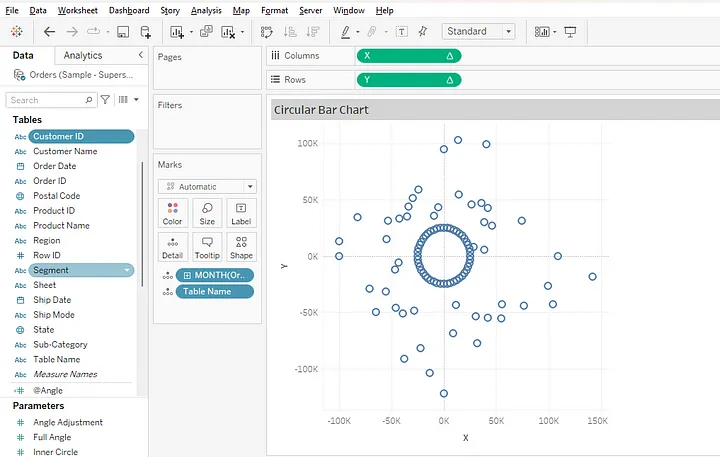top of pageSearch

# How to create a Circular Bar Chart in TableauHere is another fascinating chart in Tableau known as Circular Bar Chart. The Circular Bar Chart is wrapped around a circle. This chart is quite effective when we want to show the time series in less space in the dashboard.

History of Circular Barplot

Circular bar plots evolved out of bar charts as a substitute to represent data when required to make the visual data story look aesthetically pleasing. As humans are naturally drawn to circles and curves these charts became more popular however circular visuals also came in conflict with the effective communication of data. It tends to serve well when one’s goal is especially to present an attention-captivating chart and get an aggregate sense of the data.

## Types of Circular Bar plot

1. Stacked Circular Barplot: These charts are arranged from highest to lowest incidence.

2. Radial Barplots: A bar plot plotted on a polar coordinate system, rather than on a cartesian one.

3. Double Circular Barplot: A chart where two numeric variables are available for each group of the dataset, and are facing each other on the chart.

In this chart, we have color-coded the visualization based on the year where each line represents the sales for a month and year. The length of the line depends on the sales for that particular month. In this chart, we have two circles, the inner circle which is the starting point for the bars, and the outer circle where we are deciding how far the bars should go. Months/Years are going in the anti-clockwise direction starting from January 2018 to December 2021. So let’s dive into it to see how to create a Circular bar Chart.

Step 1 :

Connect to the Sample Superstore dataset.Step 2 : For the circular bar chart, we need two circles. One is the inner circle and the other for the length of the bars. To create two circles we have to duplicate the dataset. So will create the union of Orders table.Step 3 :

Create the Parameters

We will create the Parameters to make the chart more dynamic.

Inner radius: As our chart comprises two circles, we will create a parameter to define the radius of the inner circle.We will choose the Data type as Integer and set the current value to 25000 as we are showing sales. We can dynamically change it to any value depending on sales.

Full Angle: We will create a full angle for the inner circle.We will select the Data type as Integer and keep the default value as 360. We can dynamically change the value of the angle(270 or 180) depending on the requirement.

Step 4: Create the Calculated Fields

Index: Index Field will create the index for each row.Angle Increment: This field will give us the angle between the bars. Divide the Full Angle parameter that we have already created by the total number of records. We are using FIXED LOD to make it dynamic instead of hard-coding the value for the number of rows.Angle: Now we want the angle to be increasing continuously. For this, we will multiply the Angle Increment calculated field that we have already created with their respective index value. We will convert this value into radians as Tableau understands angles in radians.Radius: This calculated field will give the radius for the inner circle and the outer circle.X-Axis: Creating the X-AxisY-Axis: Creating the Y-AxisStep 5: Creating the Visualization

i. Drag the X to columns and Y to rows.

ii. Drag the Order Date to the details in the marks card. Then convert it to discrete and year to month.iii. Drag the Table Name to details in the marks card.

iv. Click on X and Y axis and select Compute Using Order Date.We will see that the inner circle is being created and we have outer circle points based on the sales value.v. Select the line chart from the marks card and drag the Order Date to Color. Now the Circular Bar Chart is ready.vi. Show the parameters Full Angle and Inner Circle. We can change these values and see how our chart changes shape accordingly. For example, when we change the Full Angle to 270 the chart will look as follows.When to Use a Circular Bar plot

1. When the number of bars to be represented are large: Use a circular bar plot when an obvious pattern pops out of a bar chart which has a large number of bars. This circular bar chart can become eye-catching in that case and make better use of the space than a long and usual barplot. However, since a circular bar plot is based on a general bar chart it is essential to have a good understanding of how barplot works before making it circular.

2. When your stacked bar chart looks cluttered with bars: When your stacked bar chart looks cluttered with bars: Stack circular bar charts can be used to represent multiple categories if your stacked bar chart appears cluttered with bars. They look like stacked bar charts except they have more bars. In such a case, circular bar plot can get even more interesting with a grouping variable as it becomes easy to compare groups and entities into each group. Also if the levels of your categoric variable have no obvious order, it is useful to order the bars following their values while representing in a circular plot.

3. To visualize smaller number of bars in a visually appealing way: If there are fewer bars and you want the chart to convey visual appeal, use a radial layout barplot. It is plotted using polar coordinates, but it may hinder comparison, so keep in mind that they are primarily meant to emphasize cyclical patterns. For Radial Bar Charts it is important to note that each bar on the outside gets relatively longer to the last, even if they represent the same value as each bar has to be at different radii, so each bar needs to be judged by its angle.

Conclusion

This is what Circular Bar Chart looks like for month-wise sales. As we saw that Circular Bar Chart uses circular shapes to compare key metrics in our data. The chart shares a resemblance with Bar Charts. But, they use circular bars to display insights. We can use this chart to compare the performance of key variables in our raw data. Hope this blog will help you to create the circular bar chart. Thanks for reading.